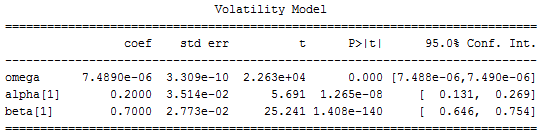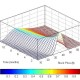## Forecasting Volatility with GARCH Model-Volatility Analysis in Python

In a previous post, we presented an example of volatility analysis using Close-to-Close historical volatility. In this post, we are going to use the Generalized Autoregressive Conditional Heteroskedasticity (GARCH) model to forecast volatility.

In econometrics, the autoregressive conditional heteroscedasticity (ARCH) model is a statistical model for time series data that describes the variance of the current error term or innovation as a function of the actual sizes of the previous time periods’ error terms; often the variance is related to the squares of the previous innovations. The ARCH model is appropriate when the error variance in a time series follows an autoregressive (AR) model; if an autoregressive moving average (ARMA) model is assumed for the error variance, the model is a generalized autoregressive conditional heteroskedasticity (GARCH) model.

ARCH models are commonly employed in modeling financial time series that exhibit time-varying volatility and volatility clustering, i.e. periods of swings interspersed with periods of relative calm. ARCH-type models are sometimes considered to be in the family of stochastic volatility models, although this is strictly incorrect since at time t the volatility is completely pre-determined (deterministic) given previous values.  Read more

As an example, we are going to apply the GARCH model to the SP500. We first downloaded 5 years of historical data of SPY from Yahoo Finance. Next, we used the first 4 years of data as the training set and fit the data to the GARCH (1, 1) model. The Python ARCH program returned the following model parameters,After obtaining the parameters, we applied the model to the remaining 1 year of data and calculated the forecasted volatility on a rolling window of 1 month. The picture below shows the rolling forecasted volatility,